# LOOKUP Excel FunctionArticle byExcelMojo Team## What Is LOOKUP Function In Excel?

The LOOKUP function in Excel is an inbuilt Lookup & Reference function. It looks up the specified value in one row or column and finds the approximate or exact match from the same position in another range of row or column.

Users get two forms of LOOKUP Excel function, vector, and array. While the vector form is useful when the search range is a single row or column, the array form works well when the search range is an array.

For example, the first table contains a list of fruits and their quantity details.

Suppose the requirement is to determine the quantity of the fruits given in the second table and display the output in cells E2:E4. Then, we can use the LOOKUP Excel function in the target cells to achieve the required data.

In the above LOOKUP Excel function example, the second table shows three entries in cells D2:D4, Orange, Oranges, and Orange. While the LOOKUP() in the first two target cells is in vector form, the one in the third target cell is in the array form.

The target cell E2 LOOKUP() performs an exact match to return the required quantity of the fruit Orange from the same position in the second column as Orange, 5500. On the other hand, the LOOKUP() in the target cell E3 does an approximate match, as the source fruits’ list contains the value Orange. And thus, the function output is 5500.

In the case of the target cell E4, the LOOKUP() searches for the value Orange in the array range A1:B6. And it finds it in cell A4 to return the value from the exact position in the last column of the array, cell B4, 5500.

###### Key Takeaways
• The LOOKUP Excel function searches for a value in one row or column to return a value from the exact position in another row or column. The match can be either exact or approximate.
• Excel offers two forms of LOOKUP(), vector and array.
• The vector form takes two mandatory arguments, lookup_value and lookup_vector, and one optional argument, result_vector, as inputs. And the array form accepts two mandatory arguments, lookup_value and array, as inputs.
• Users can use the vector form of the LOOKUP() when the search range is a single row or column and the array form when the search range is a table of rows and columns.

### LOOKUP() Excel Formula

We shall see the syntax for the two forms of LOOKUP function, vector, and array.

#### Vector Form

This form of the LOOKUP() allows us to specify the row or column containing the value to search. But we must provide the range from where we require the match for the lookup value.

The vector form LOOKUP() syntax is:

where,

• lookup_value: The value the LOOKUP Excel formula searches for in the first specified lookup vector.
• lookup_vector: A range containing only a single row or column.
• result_vector: A range with the size same as lookup_vector, containing only a single row or column.

While the first two arguments in the vector form LOOKUP() syntax are mandatory, the last argument is optional. And if we choose to ignore result_vector, the function will return the result from lookup_vector.

#### Array Form

The array form searches the lookup value in the first row or column of an array and returns the value from the exact position in the last row or column of the array.

The array form LOOKUP() syntax is:

where,

• lookup_value: The value the LOOKUP() searches for in the given array.
• array: The range the LOOKUP() compares with the lookup value.

The above arguments in the above array form LOOKUP() syntax are mandatory.

Further, adhering to the below points will ensure to avoid the condition of the LOOKUP Excel function not working.

• We can enter lookup_value as a number, text, logical value, name, or reference to the specific value.
• We can enter lookup_vector (vector form) and the array values (array form)as text, numbers, or logical values, but in ascending order. It can be A-Z if text, negative to positive numbers if numbers, FALSE and TRUE if logical values.
• Suppose the LOOKUP() cannot find the specified lookup value. Then, it matches the largest value in the lookup_vector (vector form) or array (array form), less than or equal to lookup_value.
• The LOOKUP() is case-insensitive.
• If the given array in the array form LOOKUP() covers a range containing more columns than rows, the function searches for the lookup_value in the first row.
• Suppose the given array in the array form LOOKUP() contains more rows than columns. Then the function searches for the lookup_value in the first column.
• Suppose the lookup value is smaller than the smallest value in lookup_vector (vector form) or the first row or column in the given array (array form). Or, the lookup_vector (vector form) or array (array form) is not in ascending order. Then, the function returns the #N/A error.
• Suppose we delete cells after entering the LOOKUP(), or the relative references in the LOOKUP() become invalid when we copy the function into another cell. Then, the function returns the #Ref! error.

### How To Use LOOKUP Excel Function?

The steps to apply the LOOKUP Excel Function are:

1. First, ensure that the lookup_vector (vector form) or the first row or column of the array (array form) is in ascending order.
2. Then, select the target cell and enter the LOOKUP().
3. Finally, press Enter to view the result.

The below LOOKUP example explains the above steps in detail.

The first table in the below image contains an employee list and their workstation ID details.

And suppose the requirement is to display the workstation ID in cell G2 for the given employee ID in the second table. Then we can apply the LOOKUP() in the target cell to get the required data.

#### Vector Form

• Step 1: Select the target cell G2, enter the LOOKUP(), and press Enter.

=LOOKUP(F2,A1:A11,C1:C11)

The function searches for the given lookup value, APP_115, in column A1:A11, which it finds in cell A6. And so, the function returns the value in row 6 of column C1:C11 (cell C6), 4513.

Alternatively, we can apply the LOOKUP() using the option in the Formulas tab by first selecting the target cell G2 and clicking FormulasLookup & ReferenceLOOKUP. It will open the Select Arguments window.

Next, choose the vector form option in the Select Arguments window and click OK to open the Function Arguments window.

Next, enter the LOOKUP() argument values in the Function Arguments window.

And once we click OK in the Function Arguments window, the LOOKUP() will get executed in the target cell G2.

#### Array Form

• Step 1: Select the target cell G2, enter the array form of the LOOKUP() and press Enter.

=LOOKUP(F2,A1:C11)

The function looks up the given value, APP_115, in the first column of the specified array, A1:C11, and finds the value in cell A6. Hence, it returns the value in row 6 of the last column of the array, C1:C11 (cell C6), 4513.

We may also use the LOOKUP() available in the Formulas tab. The process is the same as explained for the vector form, except you must choose the array form, the second option in the Select Arguments window.

Once we click OK, the Function Arguments window will open.

Next, enter the LOOKUP() argument values in the Function Arguments window.

Finally, click OK to see the LOOKUP() executed in the target cell, G2.

### Examples

Check out the best ways to use the LOOKUP() to avoid the conditions of the LOOKUP Excel function not working.

#### Example #1

We can apply the LOOKUP Excel function to determine the last non-empty cell in a row or column. And this example focuses on finding the required cell in a column.

The first table in the below image contains the order status of a list of items.

And suppose we need to update the last order details in the second table cells G3:G4. Then, here is how the LOOKUP function in the target cells will fetch us the required data.

• Step 1: Select the target cell G3, enter the following LOOKUP(), and press Enter.

=LOOKUP(2,1/(B:B<>””),B:B)

First, the expression (B:B<>””) checks each cell in the cell range B:B. And it returns an array of TRUEs and FALSEs, {TRUE;TRUE;TRUE;TRUE;TRUE;TRUE;TRUE;FALSE;FALSE;…}, with TRUE indicating a non-empty cell and FALSE denoting an empty cell. Then the formula divides the value 1 by each array element, with TRUE and FALSE taking the values 1 and 0. Thus, lookup_vector becomes {1;1;1;1;1;1;1;#DIV/0!;#DIV/0!;…}.

The lookup_value can be any value greater than 1, as the above LOOKUP() performs an approximate match. And the lookup value, 2, is greater than the largest value, 1, in the lookup_vector. Hence, the LOOKUP() determines the match with the last 1 in the array as the last non-empty cell. It thus returns the value in the same position in column B:B (cell B7), Samsung Galaxy S21 5G.

• Step 2: Select the target cell G4, enter the following LOOKUP(), and press Enter.

=LOOKUP(2,1/(B:B<>””),C:C)

This formula evaluates as explained in the previous step. However, the only difference is that the function looks up for the last non-empty cell in column B:B and returns the value from the same position in column C (cell C7), Order Yet To Be Dispatched.

#### Example #2

This example explains the LOOKUP Excel function array form.

The below image shows two tables. The first table contains the points secured and the corresponding grade we need to determine based on the legend provided in the second table. And the legend shows the points required to secure the specific grade.

And suppose we need to display the output in cell B2. Then apply the LOOKUP function array form in the target cell to get the secured grade.

• Step 1: Select the target cell B2, enter the LOOKUP(), and press Enter.

=LOOKUP(A2,F1:K2)

The function conducts a horizontal lookup for the value 64 in the array F1:K2. But the value 64 is not in the second table. So, the function performs an approximate match and returns the secured grade as C, as 64 is greater than the closest largest value, 55.

#### Example #3

Let us see how to use the LOOKUP Excel function with other Excel functions such as VLOOKUP.

The first table contains a list of employees and their appraisal ratings.

And suppose the requirement is to update the full forms of the ratings for the respective employees in the second table in cells G2:G11. Then, we can use the LOOKUP Excel function with VLOOKUP() in the target cells.

• Step 1: Select the target cell G2, enter the LOOKUP() containing the VLOOKUP, and press Enter.

=LOOKUP(VLOOKUP(F2,\$A\$1:\$C\$11,3,0),{“EE”;”EP”;”ME”;”UP”},{“Exceeding Expectation”;”Exceptional Performance”;”Meets Expectation”;”Under Performer”})

First, the VLOOKUP() looks up the name Benjamin Todd in the first table to return the appraisal rating, ME. Next, the LOOKUP() searches for the value ME in the lookup_vector, {“EE”;”EP”;”ME”;”UP”}. Then it returns the value from the same position in the result_vector, {“Exceeding Expectation”;”Exceptional Performance”;”Meets Expectation”;”Under Performer”}. And thus, the function output is Meets Expectation.

• Step 2: Drag the fill handle downwards to copy the formula in cells G3:G11.

### Important Things to Note

• Ensure the lookup_vector (vector form) and first row or column of the given array (array form) is in ascending order. Otherwise, the LOOKUP function will return the #N/A error.
• For a lookup_value smaller than the smallest value in the lookup_vector (vector form) or first row or column in the array (array form), the LOOKUP() returns the #N/A error.
• When we delete cells after applying the LOOKUP(), or the relative references used in the LOOKUP() become invalid, the function throws the #Ref! error.

1. Where is the LOOKUP function in Excel?

The LOOKUP function in Excel is in the Formulas tab. We can select FormulasLookup & ReferenceLOOKUP to apply it in the required cell.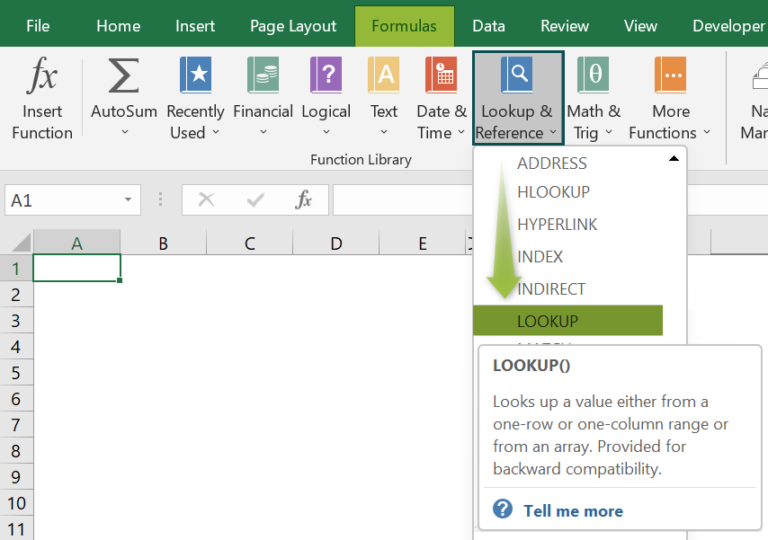2. How to use the LOOKUP and IF functions in Excel?

We can use the LOOKUP and IF functions in Excel in the following way. Let us see the steps with an example.

The first table in the below image contains the quarterly sales figures for 2020-21.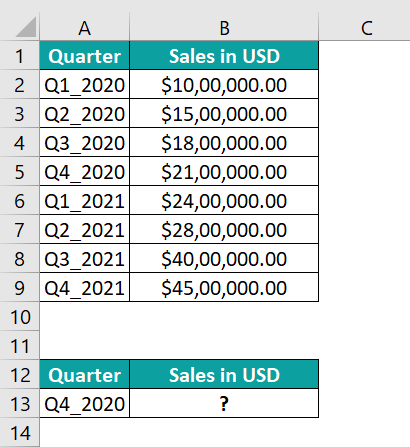And suppose the requirement is to display the specified quarter’s sales value in cell B13 of the second table. Then we can use the LOOKUP() in the IF() in the target cell to achieve the required data.

• Step 1: Select the target cell B13, enter the below formula, and press Enter.
=IF(ISBLANK(A13),”Enter A Quarter”,LOOKUP(A13,A1:A9,B1:B9))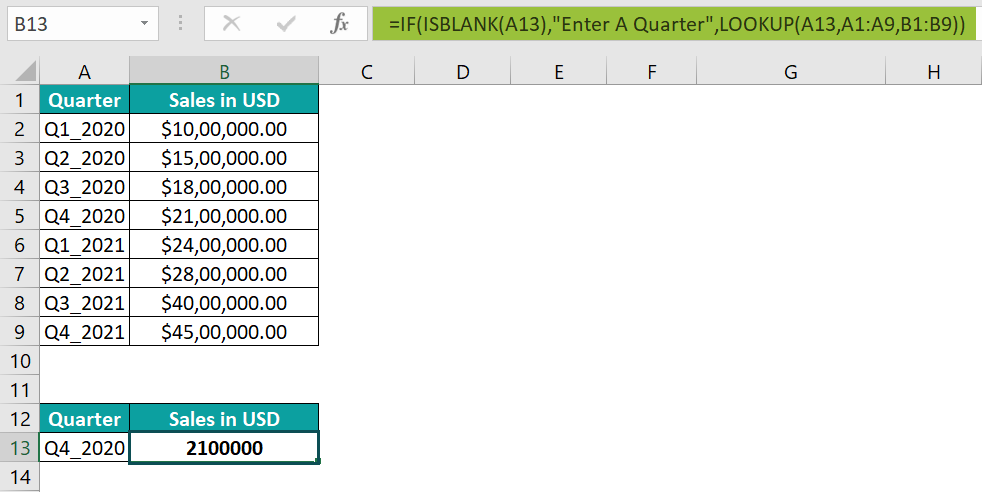First, the ISBLANK() checks if cell A13 is blank. Since it is not blank, the LOOKUP() gets executed.

It searches for the quarter value Q4_2020 in the column range, A1:A9, and finds the value in cell A5. And hence it returns the value from the same position in column B1:B9, 2100000.

• Step 2: Select cell B13 and set the data format as Currency using the Number Format option in the Home tab.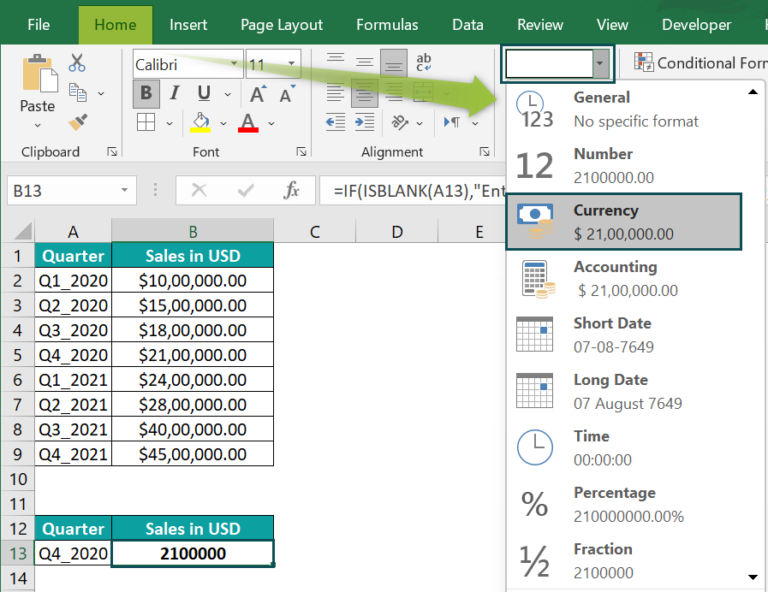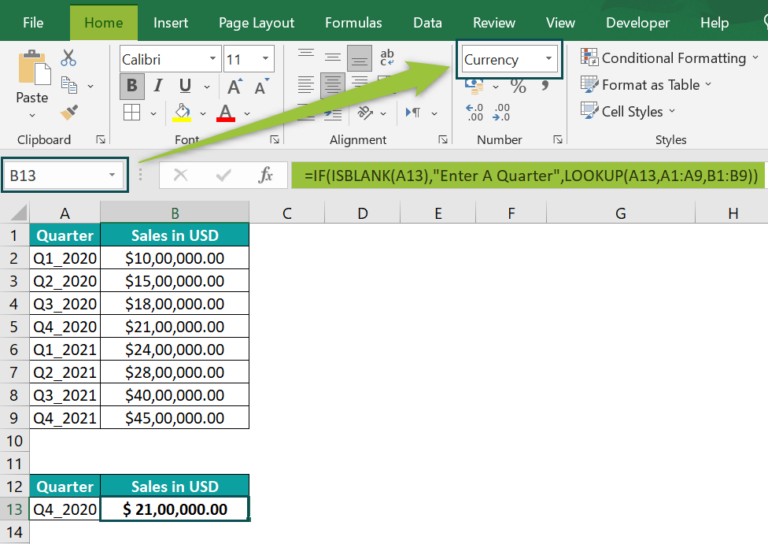3. What is the use of the LOOKUP Excel function?

The use of the LOOKUP Excel function is that it helps search for a value in a single row or column or when the search range is a set of rows and columns. And it returns a value from the same position in another row or column, even if the search match is approximate.